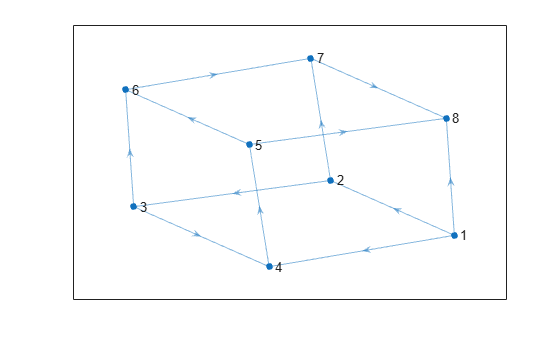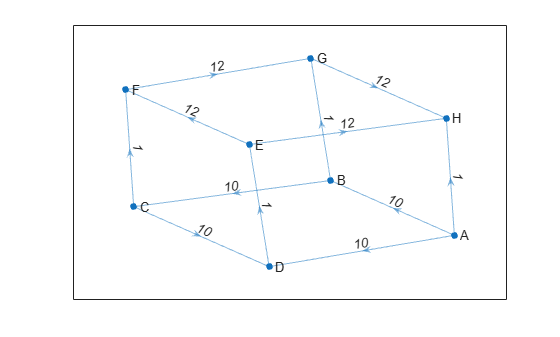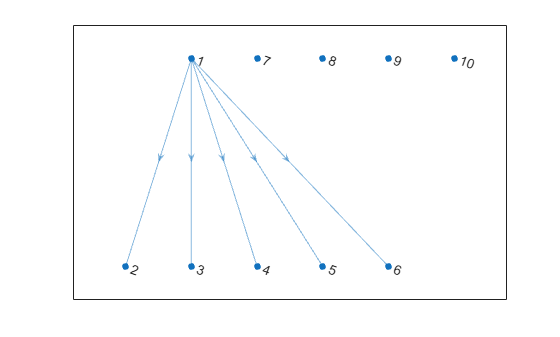# digraph

Graph with directed edges

## Description

`digraph` objects represent directed graphs, which have directional edges connecting the nodes. After you create a `digraph` object, you can learn more about the graph by using the object functions to perform queries against the object. For example, you can add or remove nodes or edges, determine the shortest path between two nodes, or locate a specific node or edge.

```G = digraph([1 1], [2 3]) e = G.Edges G = addedge(G,2,3) G = addnode(G,4) plot(G)```

## Creation

### Syntax

``G = digraph``
``G = digraph(A)``
``G = digraph(A,nodenames)``
``G = digraph(A,NodeTable)``
``G = digraph(A,___,'omitselfloops')``
``G = digraph(s,t)``
``G = digraph(s,t,weights)``
``G = digraph(s,t,weights,nodenames)``
``G = digraph(s,t,weights,NodeTable)``
``G = digraph(s,t,weights,num)``
``G = digraph(s,t,___,'omitselfloops')``
``G = digraph(s,t,EdgeTable,___)``
``G = digraph(EdgeTable)``
``G = digraph(EdgeTable,NodeTable)``
``G = digraph(EdgeTable,___,'omitselfloops')``

### Description

example

````G = digraph` creates an empty directed graph object, `G`, which has no nodes or edges.```

example

````G = digraph(A)` creates a weighted directed graph using a square adjacency matrix, `A`. The location of each nonzero entry in `A` specifies an edge for the graph, and the weight of the edge is equal to the value of the entry. For example, if ```A(2,1) = 10```, then `G` contains an edge from node 2 to node 1 with a weight of 10.```

example

````G = digraph(A,nodenames)` additionally specifies node names. The number of elements in `nodenames` must be equal to `size(A,1)`.```
````G = digraph(A,NodeTable)` specifies node names (and possibly other node attributes) using a table, `NodeTable`. The table must have the same number of rows as `A`. Specify node names using the table variable `Name`.```

example

````G = digraph(A,___,'omitselfloops')` ignores the diagonal elements of `A` and returns a graph without any self-loops. You can use any of the input argument combinations in previous syntaxes.```

example

````G = digraph(s,t)` specifies directed graph edges `(s,t)` in pairs to represent the source and target nodes. `s` and `t` can specify node indices or node names.```

example

````G = digraph(s,t,weights)` also specifies edge weights with the array `weights`.```

example

````G = digraph(s,t,weights,nodenames)` additionally specifies node names using the cell array of character vectors or string array, `nodenames`. `s` and `t` cannot contain node names that are not in `nodenames`.```
````G = digraph(s,t,weights,NodeTable)` specifies node names (and possibly other node attributes) using a table, `NodeTable`. Specify node names using the `Name` table variable. `s` and `t` cannot contain node names that are not in `NodeTable`.```

example

````G = digraph(s,t,weights,num)` specifies the number of nodes in the graph with the numeric scalar `num`.```
````G = digraph(s,t,___,'omitselfloops')` does not add any self-loops to the graph. That is, any `k` that satisfies `s(k) == t(k)` is ignored. You can use any of the input argument combinations in previous syntaxes.```
````G = digraph(s,t,EdgeTable,___)` uses a table to specify edge attributes instead of specifying `weights`. The `EdgeTable` input must be a table with a row for each corresponding pair of elements in `s` and `t`. Specify edge weights using the table variable `Weight`.```

example

````G = digraph(EdgeTable)` uses the table `EdgeTable` to define the graph. With this syntax, the first variable in `EdgeTable` must be named `EndNodes`, and it must be a two-column array defining the edge list of the graph.```

example

````G = digraph(EdgeTable,NodeTable)` additionally specifies the names (and possibly other attributes) of the graph nodes using a table, `NodeTable`.```
````G = digraph(EdgeTable,___,'omitselfloops')` does not add self-loops to the graph. That is, any `k` that satisfies ```EdgeTable.EndNodes(k,1) == EdgeTable.EndNodes(k,2)``` is ignored. You must specify `EdgeTable` and optionally can specify `NodeTable`.```

### Input Arguments

expand all

Adjacency matrix, specified as a full or sparse, numeric matrix. The entries in `A` specify the network of connections (edges) between the nodes of the graph. The location of each nonzero entry in `A` specifies an edge between two nodes. The value of that entry provides the edge weight. A logical adjacency matrix results in an unweighted graph.

Nonzero entries on the main diagonal of `A` specify self-loops, or nodes that are connected to themselves with an edge. Use the `'omitselfloops'` input option to ignore diagonal entries.

Example: `A = [0 1 0; 0 0 0; 5 0 0]` describes a graph with three nodes and two edges. The edge from node 1 to node 2 has a weight of `1`, and the edge from node 3 to node 1 has a weight of `5`.

Data Types: `single` | `double` | `logical`

Node names, specified as a cell array of character vectors or string array. `nodenames` must have length equal to `numnodes(G)` so that it contains a nonempty, unique name for each node in the graph.

Example: `G = digraph(A,{'n1','n2','n3'})` specifies three node names for a 3-by-3 adjacency matrix, `A`.

Data Types: `cell` | `string`

Source and target node pairs, specified as node indices or node names. `digraph` creates directed edges between the corresponding nodes in `s` and `t`, which must both be numeric, or both be character vectors, cell arrays of character vectors, categorical arrays, or string arrays. In all cases, `s` and `t` must have the same number of elements.

• If `s` and `t` are numeric, then they correspond to indices of graph nodes. Numeric node indices must be positive integers greater than or equal to 1.

• If `s` and `t` are character vectors, cell arrays of character vectors, or string arrays, then they specify names for the nodes. The `Nodes` property of the graph is a table containing a `Name` variable with the node names, `G.Nodes.Name`.

• If `s` and `t` are categorical arrays, then the categories in `s` and `t` are used as the node names in the graph. This can include categories that are not elements in `s` or `t`.

• If `s` and `t` specify multiple edges between the same two nodes, then the result is a multigraph.

This table shows the different ways to refer to one or more nodes either by their numeric node indices or by their node names.

FormSingle NodeMultiple Nodes
Node index

Scalar

Example: `1`

Vector

Example: `[1 2 3]`

Node name

Character vector

Example: `'A'`

Cell array of character vectors

Example: `{'A' 'B' 'C'}`

String scalar

Example: `"A"`

String array

Example: `["A" "B" "C"]`

Categorical array

Example: `categorical("A")`

Categorical array

Example: `categorical(["A" "B" "C"])`

Example: `G = digraph([1 2 3],[2 4 5])` creates a graph with five nodes and three edges.

Example: ```G = digraph({'Boston' 'New York' 'Washington D.C.'},{'New York' 'New Jersey' 'Pittsburgh'})``` creates a graph with five named nodes and three edges.

Edge weights, specified as a scalar, vector, matrix, or multidimensional array. `weights` must be a scalar or an array with the same number of elements as `s` and `t`.

`digraph` stores the edge weights as a `Weight` variable in the `G.Edges` property table. To add or change weights after creating a graph, you can modify the table variable directly, for example, ```G.Edges.Weight = [25 50 75]'```.

If you specify `weights` as an empty array `[]`, then it is ignored.

Example: `G = digraph([1 2],[2 3],[100 200])` creates a graph with three nodes and two edges. The edges have weights of `100` and `200`.

Data Types: `single` | `double`

Number of graph nodes, specified as a positive scalar integer. `num` must be greater than or equal to the largest elements in `s` and `t`.

Example: `G = digraph([1 2],[2 3],[],5)` creates a graph with three connected nodes and two isolated nodes.

Table of edge information. If you do not specify `s` and `t`, then the first variable in `EdgeTable` is required to be a two-column matrix, cell array of character vectors, or string array called `EndNodes` that defines the graph edges. For edge weights, use the variable `Weight`, since this table variable name is used by some graph functions. If there is a variable `Weight`, then it must be a numeric column vector. See `table` for more information on constructing a table.

After creating a graph, query the edge information table using `G.Edges`.

Example: ```EdgeTable = table([1 2; 2 3; 3 5; 4 5],'VariableNames',{'EndNodes'})```

Data Types: `table`

Table of node information. `NodeTable` can contain any number of variables to describe attributes of the graph nodes. For node names, use the variable `Name`, since this variable name is used by some graph functions. If there is a variable `Name`, then it must be a cell array of character vectors or string array specifying a unique name in each row. See `table` for more information on constructing a table.

After the graph is created, query the node information table using `G.Nodes`.

Example: ```NodeTable = table({'a'; 'b'; 'c'; 'd'},'VariableNames',{'Name'})```

Data Types: `table`

### Output Arguments

expand all

Directed graph, returned as a `digraph` object.

## Properties

expand all

Edges of graph, returned as a table. By default this is an `M`-by-`1` table, where `M` is the number of edges in the graph.

• To add new edge properties to the graph, create a new variable in the `Edges` table.

• To add or remove edges from the graph, use the `addedge` or `rmedge` object functions.

Example: `G.Edges` returns a table listing the edges in the graph

Example: `G.Edges.Weight` returns a numeric vector of the edge weights.

Example: `G.Edges.Weight = [10 20 30 55]'` specifies new edge weights for the graph.

Example: ```G.Edges.NormWeight = G.Edges.Weight/sum(G.Edges.Weight)``` adds a new edge property to the table containing the normalized weights of the edges.

Data Types: `table`

Nodes of graph, returned as a table. By default this is an empty `N`-by-`0` table, where `N` is the number of nodes in the graph.

• To add new node properties to the graph, create a new variable in the `Nodes` table.

• To add or remove nodes from the graph, use the `addnode` or `rmnode` object functions.

Example: `G.Nodes` returns a table listing the node properties of the graph. This table is empty by default.

Example: ```G.Nodes.Names = {'Montana', 'New York', 'Washington', 'California'}'``` adds node names to the graph by adding the variable `Names` to the `Nodes` table.

Example: `G.Nodes.WiFi = logical([1 0 0 1 1]')` adds the variable `WiFi` to the `Nodes` table. This property specifies that certain airports have wireless internet coverage.

Data Types: `table`

## Object Functions

expand all

 `addedge` Add new edge to graph `rmedge` Remove edge from graph `flipedge` Reverse edge directions `addnode` Add new node to graph `rmnode` Remove node from graph `findedge` Locate edge in graph `findnode` Locate node in graph `numedges` Number of edges in graph `numnodes` Number of nodes in graph `edgecount` Number of edges between two nodes `reordernodes` Reorder graph nodes `subgraph` Extract subgraph
 `bfsearch` Breadth-first graph search `dfsearch` Depth-first graph search `centrality` Measure node importance `toposort` Topological order of directed acyclic graph `transclosure` Transitive closure `transreduction` Transitive reduction `isdag` Determine if graph is acyclic `conncomp` Connected graph components `condensation` Graph condensation `maxflow` Maximum flow in graph `isisomorphic` Determine whether two graphs are isomorphic `isomorphism` Compute isomorphism between two graphs `ismultigraph` Determine whether graph has multiple edges `simplify` Reduce multigraph to simple graph
 `shortestpath` Shortest path between two single nodes `shortestpathtree` Shortest path tree from node `distances` Shortest path distances of all node pairs
 `adjacency` Graph adjacency matrix `incidence` Graph incidence matrix
 `indegree` In-degree of nodes `outdegree` Out-degree of nodes `predecessors` Node predecessors `successors` Node successors `nearest` Nearest neighbors within radius `inedges` Incoming edges to node `outedges` Outgoing edges from node
 `plot` Plot graph nodes and edges

## Examples

collapse all

Create a `digraph` object with three nodes and three edges. One edge is from node 1 to node 2, another is from node 1 to node 3, and the third is from node 2 to node 1.

`G = digraph([1 1 2],[2 3 1])`
```G = digraph with properties: Edges: [3x1 table] Nodes: [3x0 table] ```

View the edge table of the graph. For directed graphs, the first column indicates the source nodes of each edge, and the second column indicates the target nodes.

`G.Edges`
```ans=3×1 table EndNodes ________ 1 2 1 3 2 1 ```

Add node names to the graph, then view the new node and edge tables. The source and target nodes of each edge are now expressed using their node names.

```G.Nodes.Name = {'A' 'B' 'C'}'; G.Nodes```
```ans=3×1 table Name _____ {'A'} {'B'} {'C'} ```
`G.Edges`
```ans=3×1 table EndNodes ______________ {'A'} {'B'} {'A'} {'C'} {'B'} {'A'} ```

You can add or modify extra variables in the `Nodes` and `Edges` tables to describe attributes of the graph nodes or edges. However, you cannot directly change the number of nodes or edges in the graph by modifying these tables. Instead, use the `addedge`, `rmedge`, `addnode`, or `rmnode` functions to modify the number of nodes or edges in a graph.

For example, add an edge to the graph between nodes 2 and 3 and view the new edge list.

`G = addedge(G,2,3)`
```G = digraph with properties: Edges: [4x1 table] Nodes: [3x1 table] ```
`G.Edges`
```ans=4×1 table EndNodes ______________ {'A'} {'B'} {'A'} {'C'} {'B'} {'A'} {'B'} {'C'} ```

Create a symmetric adjacency matrix, `A`, that creates a complete directed graph of order 4. Use a logical adjacency matrix to create a graph without weights.

`A = ones(4) - diag([1 1 1 1])`
```A = 4×4 0 1 1 1 1 0 1 1 1 1 0 1 1 1 1 0 ```
`G = digraph(A~=0)`
```G = digraph with properties: Edges: [12x1 table] Nodes: [4x0 table] ```

View the edge list of the graph.

`G.Edges`
```ans=12×1 table EndNodes ________ 1 2 1 3 1 4 2 1 2 3 2 4 3 1 3 2 3 4 4 1 4 2 4 3 ```

```A = magic(4); A(A>10) = 0```
```A = 4×4 0 2 3 0 5 0 10 8 9 7 6 0 4 0 0 1 ```

Create a graph with named nodes using the adjacency matrix. Specify `'omitselfloops'` to ignore the entries on the diagonal of `A`.

```names = {'alpha' 'beta' 'gamma' 'delta'}; G = digraph(A,names,'omitselfloops')```
```G = digraph with properties: Edges: [8x2 table] Nodes: [4x1 table] ```

View the edge and node information.

`G.Edges`
```ans=8×2 table EndNodes Weight ______________________ ______ {'alpha'} {'beta' } 2 {'alpha'} {'gamma'} 3 {'beta' } {'alpha'} 5 {'beta' } {'gamma'} 10 {'beta' } {'delta'} 8 {'gamma'} {'alpha'} 9 {'gamma'} {'beta' } 7 {'delta'} {'alpha'} 4 ```
`G.Nodes`
```ans=4×1 table Name _________ {'alpha'} {'beta' } {'gamma'} {'delta'} ```

Create and plot a cube graph using a list of the end nodes of each edge.

```s = [1 1 1 2 2 3 3 4 5 5 6 7]; t = [2 4 8 3 7 4 6 5 6 8 7 8]; G = digraph(s,t)```
```G = digraph with properties: Edges: [12x1 table] Nodes: [8x0 table] ```
`plot(G,'Layout','force')`Create and plot a cube graph using a list of the end nodes of each edge. Specify node names and edge weights as separate inputs.

```s = [1 1 1 2 2 3 3 4 5 5 6 7]; t = [2 4 8 3 7 4 6 5 6 8 7 8]; weights = [10 10 1 10 1 10 1 1 12 12 12 12]; names = {'A' 'B' 'C' 'D' 'E' 'F' 'G' 'H'}; G = digraph(s,t,weights,names)```
```G = digraph with properties: Edges: [12x2 table] Nodes: [8x1 table] ```
`plot(G,'Layout','force','EdgeLabel',G.Edges.Weight)`Create a weighted graph using a list of the end nodes of each edge. Specify that the graph should contain a total of 10 nodes.

```s = [1 1 1 1 1]; t = [2 3 4 5 6]; weights = [5 5 5 6 9]; G = digraph(s,t,weights,10)```
```G = digraph with properties: Edges: [5x2 table] Nodes: [10x0 table] ```

Plot the graph. The extra nodes are disconnected from the primary connected component.

`plot(G)`Create an empty digraph object, `G`.

`G = digraph;`

Add three nodes and three edges to the graph. The corresponding entries in `s` and `t` define the source and target nodes of the edges. `addedge` automatically adds the appropriate nodes to the graph if they are not already present.

```s = [1 2 1]; t = [2 3 3]; G = addedge(G,s,t)```
```G = digraph with properties: Edges: [3x1 table] Nodes: [3x0 table] ```

View the edge list. Each row describes an edge in the graph.

`G.Edges`
```ans=3×1 table EndNodes ________ 1 2 1 3 2 3 ```

For the best performance, construct graphs all at once using a single call to `digraph`. Adding nodes or edges in a loop can be slow for large graphs.

Create an edge table that contains the variables `EndNodes`, `Weight`, and `Code`. Then create a node table that contains the variables `Name` and `Country`. The variables in each table specify properties of the graph nodes and edges.

```s = [1 1 1 2 2 3]; t = [2 3 4 3 4 4]; weights = [6 6.5 7 11.5 12 17]'; code = {'1/44' '1/49' '1/33' '44/49' '44/33' '49/33'}'; EdgeTable = table([s' t'],weights,code, ... 'VariableNames',{'EndNodes' 'Weight' 'Code'})```
```EdgeTable=6×3 table EndNodes Weight Code ________ ______ _________ 1 2 6 {'1/44' } 1 3 6.5 {'1/49' } 1 4 7 {'1/33' } 2 3 11.5 {'44/49'} 2 4 12 {'44/33'} 3 4 17 {'49/33'} ```
```names = {'USA' 'GBR' 'DEU' 'FRA'}'; country_code = {'1' '44' '49' '33'}'; NodeTable = table(names,country_code,'VariableNames',{'Name' 'Country'})```
```NodeTable=4×2 table Name Country _______ _______ {'USA'} {'1' } {'GBR'} {'44'} {'DEU'} {'49'} {'FRA'} {'33'} ```

Create a graph using the node and edge tables. Plot the graph using the country codes as node and edge labels.

```G = digraph(EdgeTable,NodeTable); plot(G,'NodeLabel',G.Nodes.Country,'EdgeLabel',G.Edges.Code)```## Compatibility Considerations

expand all

Behavior changed in R2018a